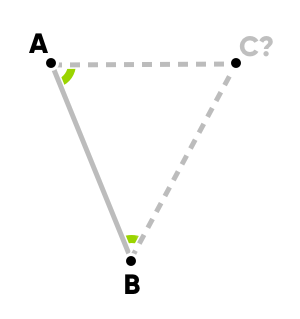# Finding third corner in triangle

#1

Maths people, rejoice! I have a conundrum for you.

If point A and B are known, as well as the angles in green, how can point C be calculated?One option is to extend the lines an arbitrarily guessed amount from A and B towards C and do a “where do these lines intersect” type affair. Is there a more elegant approach?

0 Likes

#2

I propose a solution. It is not a plug-and-play formula, I don’t know a formula for this problem and math is not a list of formulas. So try to follow the various points.

1. Applying a translation we may assume A = (0,0), once the solution is found we will apply the inverse translation to the solution.
2. Applying a rotation we may assume B = (d, 0), once the solution is found we will apply the inverse rotation to the solution.

(These two preliminary steps are usually referred to “in a suitable coordinate system…”)

1. Now the point C is the intersection of the line

y = tg(a) x

where a is the angle in A, and the other line

y = -tg(b) (x - d)

where b is the angle in B.

1. The intersection of the two lines is computed by solving a system of the two equations defining the lines. If I am not wrong, you get

x_3 = d / (tg(b) - tg(a))
y_3 = tg(a) x_3.

We now go back to the original coordinate system.

1. Apply the inverse rotation.
2. Apply the inverse translation.

A translation is simply a sum of vectors. Rotation by an angle of a radians is a multiplication by a 2x2 matrix like

cos(a) -sin(a)
sin(a) cos(a)

For the inverse put -a instead of a.

I hope this is somehow clear. If you need some more details please ask.

Ciao!

2 Likes

#3

I have another solution, maybe you like this one more than the first.

Let d be the length of AB. Let M be the projection of C to AB and let a be the length of AM and b that of MB. Then, using trigonometry, we find

a = tg(b) d / ( tg(a) + tg(b) )

b = tg(a) d / ( tg(a) + tg(b) ).

Using a and b we find the point M as

M = (b A + a B) / (a + b).

Finally the length h of MC is

h = tg(a) tg(b) d / ( tg(a) + tg(b) ).

The point C is now obtained by adding to M a vector w orthogonal to v = AB with length h. To find the orthogonal vector w first we compute v = (x_B - x_A, y_B - y_A), then we normalize it u = v / |v| , let say we get u = (x, y), and we have w = (-y, x). So

C = M + h w.

This new solution is more geometrical. I have however to say that your question hasn’t a direct solution; or at least I don’t see it…

Ciao!

2 Likes

#4

Some quick calculation. I am not really sure.
AC = AB / (cos B * (tan B / tan A + 1))

3 Likes

#5

This is right!

0 Likes

#6

Thanks guys! As much as most this is beyond my understanding, it’s fascinating and I’ll try to make sense of it.0 Likes

#7

@totebo here it is a lua code version of the second solution; after some simplification. I have not tested it, try at your risk``````local function compute_vertex(xA, yA, a, xB, yB, b)
local t = math.tan(a) + math.tan(b)
if math.abs(t) < 0.000001 then
-- the two sides of the triangles are "almost" parallel
-- and we don't want to divide by 0
return nil
end
local ma = math.tan(b) / t
local mb = math.tan(a) / t
local xM = mb * xA + ma * xB
local yM = mb * yA + ma * yB
local h = math.tan(a) * math.tan(b) / t
local xV = xB - xA
local yV = yB - yA
local xC = xM - h * yV
local yC = yM + h * xV
return xC, yC
end``````
2 Likes

#8Now, THIS is something that’s closer to home! Thanks so much! Will be a joy to try this out.

0 Likes